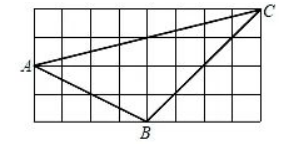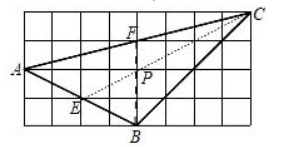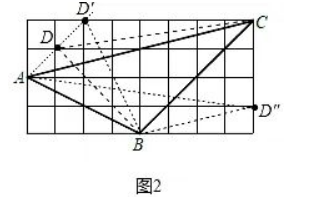(1) 画出 $\triangle A B C$ 的重心 $P$.
（2）在已知网格中找出所有格点 $D$, 使点 $D$ 与 $\triangle A B C$ 的其中两个顶点构成的三角形的面积与 $\triangle A B C$ 的面 积相等（2）如图2中，点D，D′，D″即为所求．